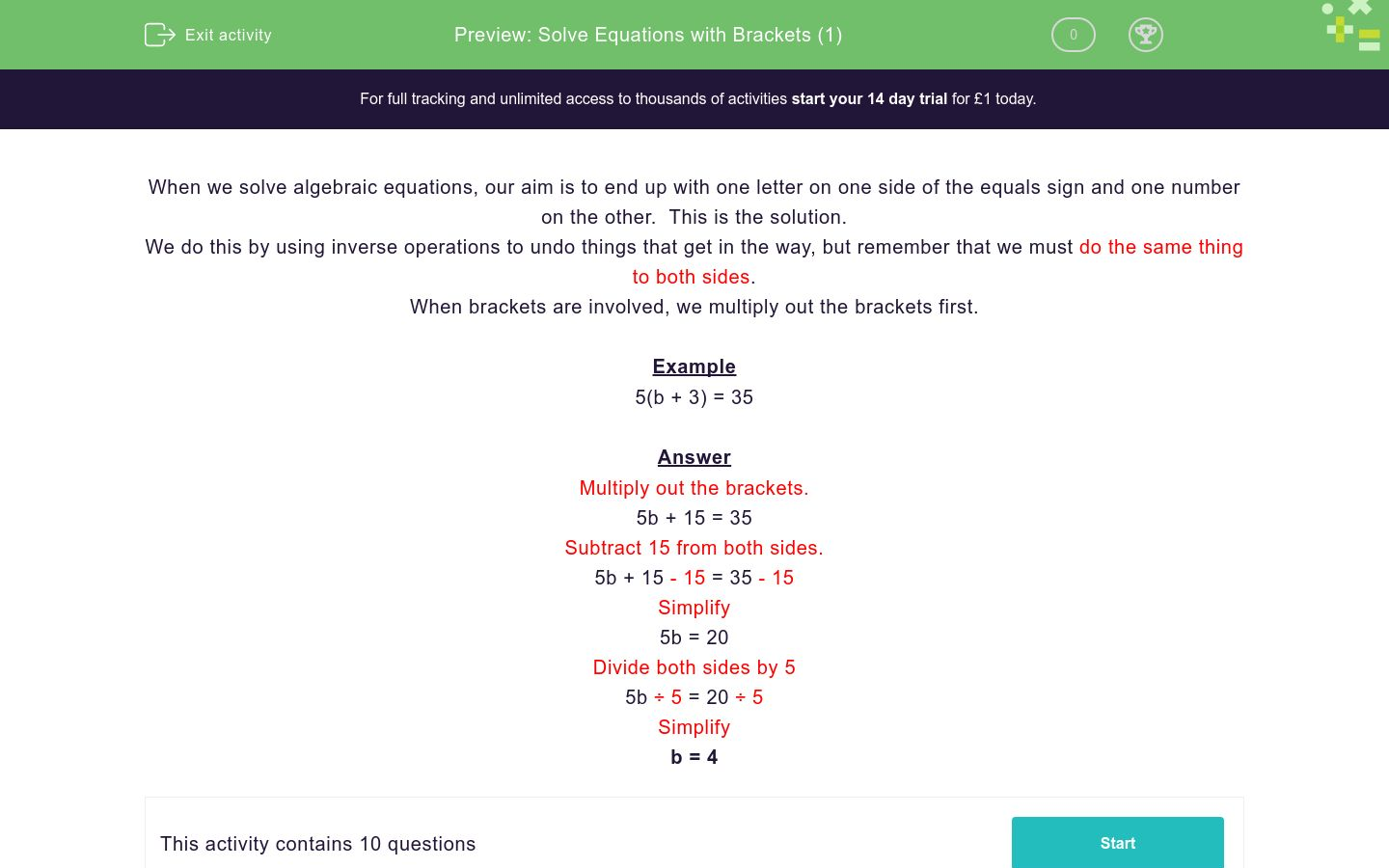# Solve Equations with Brackets (1)

In this worksheet, students solve equations with brackets.Key stage:

Curriculum topic:

Curriculum subtopic:

Difficulty level:### QUESTION 1 of 10

When we solve algebraic equations, our aim is to end up with one letter on one side of the equals sign and one number on the other.  This is the solution.

We do this by using inverse operations to undo things that get in the way, but remember that we must do the same thing to both sides.

When brackets are involved, we multiply out the brackets first.

Example

5(b + 3) = 35

Multiply out the brackets.

5b + 15 = 35

Subtract 15 from both sides.

5b + 15 - 15 = 35 - 15

Simplify

5b = 20

Divide both sides by 5

5b ÷ 5 = 20 ÷ 5

Simplify

b = 4

Solve for a:

2(a + 4) = 38

a = 17

a = 19

a = 15

Solve for a:

3(a - 4) = -3

a = 27

a = -⅓

a = 3

Solve for a:

2(4a + 1) = 34

a = 4

a = -4

a = 28

Solve for a:

2(2a - 1) = -22

a = -5

a = 24

a = -6

Solve for a:

3(a + 3) = 27

a = 9

a = 2

a = 6

Solve for a:

5(a - 4)  = 55

a = 15

a = 3

a = 59

Solve for a:

3(2 - a) = 9

a = 8

a = -2

a = -1

Solve for a:

10(2a + 3) = 130

a = -6

a = 18

a = 5

Solve for a:

5(3 - a) = 20

a = -5

a = -1

a = 1

Solve for a:

-5(3 - a) = -20

a = -1

a = ½

a = 1

• Question 1

Solve for a:

2(a + 4) = 38

a = 15
EDDIE SAYS

Multiply out the brackets.

2a + 8 = 38

Subtract 8 from both sides.

2a + 8 - 8 = 38 - 8

Simplify

2a = 30

Divide both sides by 2

2a ÷ 2 = 30 ÷ 2

Solve for a

a = 15

• Question 2

Solve for a:

3(a - 4) = -3

a = 3
EDDIE SAYS

Multiply out the brackets.

3a - 12 = -3

3a - 12 + 12 = -3 + 12

Simplify

3a = 9

Divide both sides by 3

3a ÷ 3 = 9 ÷ 3

Solve for a

a = 3

• Question 3

Solve for a:

2(4a + 1) = 34

a = 4
EDDIE SAYS

Multiply out the brackets.

8a + 2 = 34

Subtract 2 from both sides.

8a + 2 - 2 = 34 - 2

Simplify

8a = 32

Divide both sides by 8

8a ÷ 8 = 32 ÷ 8

Solve for a

a = 4

• Question 4

Solve for a:

2(2a - 1) = -22

a = -5
EDDIE SAYS

Multiply out the brackets.

4a - 2 = -22

4a - 2 + 2 = -22 + 2

Simplify

4a = -20

Divide both sides by 4

4a ÷ 4 = -20 ÷ 4

Solve for a

a = -5

• Question 5

Solve for a:

3(a + 3) = 27

a = 6
EDDIE SAYS

Multiply out the brackets.

3a + 9 = 27

Subtract 9 from both sides.

3a + 9 - 9 = 27 - 9

Simplify

3a = 18

Divide both sides by 3

3a ÷ 3 = 18 ÷ 3

Solve for a

a = 6

• Question 6

Solve for a:

5(a - 4)  = 55

a = 15
EDDIE SAYS

Multiply out the brackets.

5a - 20 = 55

5a - 30 + 20 = 55 + 20

Simplify

5a = 75

Divide both sides by 5

5a ÷ 5 = 75 ÷ 5

Solve for a

a = 15

• Question 7

Solve for a:

3(2 - a) = 9

a = -1
EDDIE SAYS

Multiply out the brackets.

6 - 3a = 9

subtract 6 from both sides.

6 - 3a - 6 = 9 - 6

Simplify

-3a = 3

Divide both sides by -3

-3a ÷ 3  = 3  ÷ -3

Solve for a

a = -1

• Question 8

Solve for a:

10(2a + 3) = 130

a = 5
EDDIE SAYS

Multiply out the brackets.

20a + 30 = 130

subtract 30 from both sides.

20a + 30 - 30 = 130 - 30

Simplify

20a = 100

Divide both sides by 20

20a ÷ 20  = 100 ÷ 20

Solve for a

a = 5

• Question 9

Solve for a:

5(3 - a) = 20

a = -1
EDDIE SAYS

Multiply out the brackets.

15 - 5a = 20

subtract 15 from both sides.

15 - 5a - 15 = 20 - 15

Simplify

-5a = 5

Divide both sides by -5

-5a ÷ -5  = 5 ÷ -5

Solve for a

a = -1

• Question 10

Solve for a:

-5(3 - a) = -20

a = -1
EDDIE SAYS

Multiply out the brackets.

-15 + 5a = - 20

-15 + 5a + 15 = -20 + 15

Simplify

5a = -5

Divide both sides by 5

5a ÷ 5 = -5 ÷ 5

Solve for a

a = -1

---- OR ----

Sign up for a £1 trial so you can track and measure your child's progress on this activity.

### What is EdPlace?

We're your National Curriculum aligned online education content provider helping each child succeed in English, maths and science from year 1 to GCSE. With an EdPlace account you’ll be able to track and measure progress, helping each child achieve their best. We build confidence and attainment by personalising each child’s learning at a level that suits them.

Get started